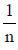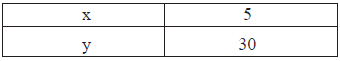# Direct And Inverse Proportions - MCQ

## 10 Questions MCQ Test Class 8 Mathematics by VP Classes | Direct And Inverse Proportions - MCQ

Description
Attempt Direct And Inverse Proportions - MCQ | 10 questions in 20 minutes | Mock test for Class 8 preparation | Free important questions MCQ to study Class 8 Mathematics by VP Classes for Class 8 Exam | Download free PDF with solutions
QUESTION: 1

Solution:
QUESTION: 2

Solution:
QUESTION: 3

### If ‘A’ can finish a work in‘n’ days then part of work finished in 1 day is:

Solution:
QUESTION: 4

If amount of work completed by ‘A’ in one day isthen the whole work will be finished by ‘A’ is:

Solution:

The part of work done by A in one day = 1/n

i.e. 1 day = 1/n part

n days = 1 part

∵ whole work = 1 part of total work,

Thus, the time taken by A in whole work would be n days.

QUESTION: 5

If an increase in one quantity brings about a corresponding decrease in the other and ice versa,then the two quantities vary:

Solution:
QUESTION: 6

“If speed is more than time to cover a fixed distance would be less”. This is a case of :

Solution:
QUESTION: 7

Which of the following is a case of inverse variation?

Solution:
QUESTION: 8

Which of the following is not a case of direct variation?

Solution:
QUESTION: 9

Which of the following is case of direct variation:

Solution:
QUESTION: 10

If x and y vary inversely. Then using the following table?The value of x for y = 10 is

Solution:Use Code STAYHOME200 and get INR 200 additional OFF Use Coupon Code